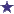# How do you figure the days supply for a liquid (syrup) medication?(Cough or Other) Syrup Medication - Days Supply

120ML: 10ml every 8 hours-How many days supply is that?

### Comments for How do you figure the days supply for a liquid (syrup) medication?

Average RatingApr 21, 2017 RatingSyrup Days Supply: Four Days of Medication by: David How much medication do we have? 120ML How often is it given/taken? Every 8 hours (or three times daily, for the purposes of this problem.) How much is the dose? 10ML We start by figuring the total maximum amount of medication that can be given/taken in one day. We figure this by multiplying (How much is the dose?) 10ML BY (How often is it given/taken?) Three times daily = 30ML/DAY Now we divide the total amount (How much medication do we have?) 120ML by (the maximum amount of medication given/taken in one day) 30ML/DAY which equals 4. The answer is: 120ML of Cough Syrup, given/taken at 10ML every 8 hours will have a days supply of FOUR DAYS.